# Notes on BRST IV: Lie Algebra Cohomology for Semi-simple Lie Algebras

In this posting I’ll work out some examples of Lie algebra cohomology, still for finite dimensional Lie algebras and representations.

Ifis a compact, connected Lie group, it can be thought of as a compact manifold, and as such one can define its de Rham cohomology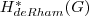as the cohomology of the complex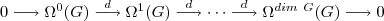where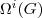are the differential i-forms on(note, we’ll use complex-valued forms), and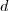is the deRham differential.

For a compact group, one has a bi-invariant Haar measure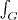, and can use this to “average” over an action of the group on a space. For a representation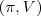, we get a projection operator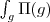onto the invariant subspace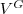. This projection operator gives explicitly the invariants functor on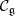. It is an exact functor, taking exact sequences to exact sequences.

The differential forms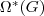give a representation ofin two ways, taking the induced action on forms by pullback, using either left or right translation on the group. If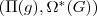is the representation by left translations, we can use this to apply our “averaging over” projection operator to the de Rham complex. This action commutes with the de Rham differential, so we get a sub-complex of left-invariant forms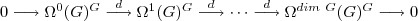Since elements of the Lie algebra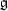are precisely left-invariant 1-forms, it turns out that this complex is nothing but the Chevalley-Eilenberg complex considered last time to represent Lie algebra cohomology, for the case of the trivial representation. This means we have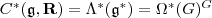, and the differentials coincide. So, what we have shown is that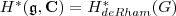If one knows the cohomology of, the Lie algebra cohomology is thus known, but this identity is normally used in the other direction, to find the cohomology offrom that of the Lie algebra. To compute the Lie-algebra cohomology, we can exploit the right-action of G on the group, averaging over the induced action on the left-invariant forms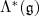, which again commutes with the differential. We end up with a complex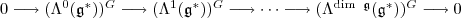where all the differentials are zero, so the cohomology is given by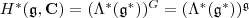the adjoint-invariant pieces of the exterior algebra on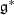. Finding the cohomology has now been turned into a purely algebraic problem in invariant theory. For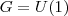,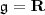, and we have shown that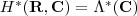, this is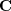in degrees 0, and 1, as expected for the de Rham cohomology of the circle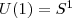. For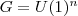, we get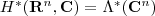Note that complexifying the Lie algebra and working with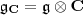commutes with taking cohomology, so we get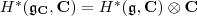Complexifying the Lie algebra of a compact semi-simple Lie group gives a complex semi-simple Lie algebra, and we have now computed the cohomology of these as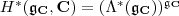Besides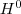, one always gets a non-trivial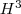, since one can use the Killing formto produce an adjoint-invariant 3-form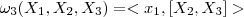. For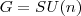,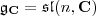, and one gets non-trivial cohomology classes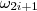for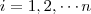, such that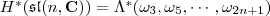the exterior algebra generated by the.

To compute Lie algebra cohomology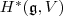with coefficients in a representation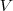, we can go through the same procedure as above, starting with differential forms ontaking values in, or we can just use exactness of the averaging functor that takesto. Either way, we end up with the result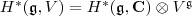Thepiece of this is just the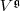that we want when we are doing BRST, but we also get quite a bit else: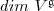copies of the higher degree pieces of the Lie algebra cohomology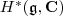. The Lie algebra cohomology here is quite non-trivial, but doesn’t interact in a non-trivial way with the process of identifying the invariantsin.

In the next posting I’ll turn to an example where Lie algebra cohomology interacts in a much more interesting way with the representation theory, this will be the highest-weight theory of representations, in a cohomological interpretation first studied by Bott and Kostant.

Last Updated on

This entry was posted in BRST. Bookmark the permalink.

### 2 Responses to Notes on BRST IV: Lie Algebra Cohomology for Semi-simple Lie Algebras

1. Anon says:

I’m a bit confused by the step where the De Rham complex of differential forms on the group G is found to have the same cohomology as the complex of left-invariant differential forms. Did I miss the step where this was explained above?

2. Peter Woit says:

Anon,

I guess maybe I’m skipping some steps there…

The argument is that:

1. Because the averaging functor is exact, the cohomology of the complex with terms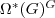is2. But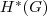is-invariant, since it is homotopy-invariant, andis connected.